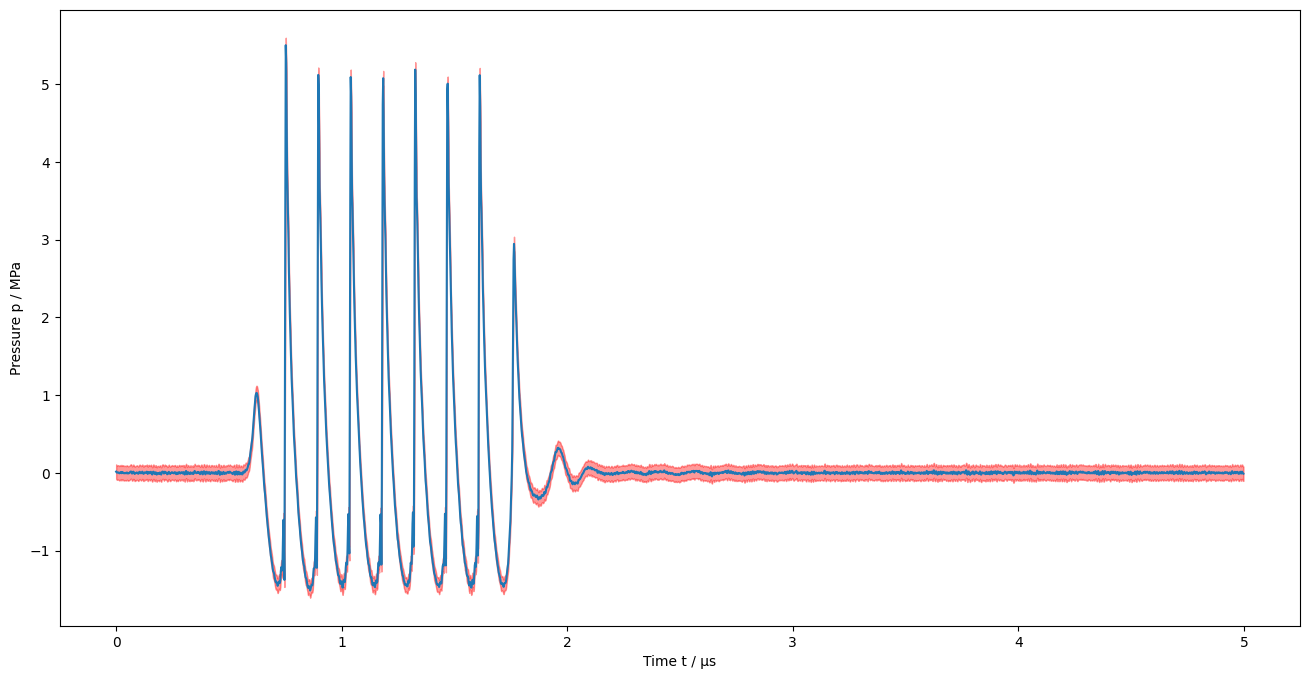# 5. Deconvolution in the frequency domain¶

:

%pylab inline

Populating the interactive namespace from numpy and matplotlib

:

from meas_data_preprocessing import *
from hydrophone_data_preprocessing import *
from PyDynamic.uncertainty.propagate_DFT import DFT_deconv, GUM_iDFT

/home/ludwig10/code/envs/PyDynamic_tutorials-py38/lib/python3.8/site-packages/PyDynamic/identification/fit_filter.py:24: DeprecationWarning: The module *identification* will be combined with the module *deconvolution* and renamed to *model_estimation* in the next major release 2.0.0. From version 1.4.1 on you should only use the new module *model_estimation* instead.
warnings.warn(
/home/ludwig10/code/envs/PyDynamic_tutorials-py38/lib/python3.8/site-packages/PyDynamic/identification/fit_transfer.py:23: DeprecationWarning: The package *identification* will be combined with the package *deconvolution* and renamed to *model_estimation* in the next major release 2.0.0. From version 1.4.1 on you should only use the new package *model_estimation* instead.
warnings.warn(
/home/ludwig10/code/envs/PyDynamic_tutorials-py38/lib/python3.8/site-packages/PyDynamic/uncertainty/interpolation.py:24: PendingDeprecationWarning: The module :mod:PyDynamic.uncertainty.interpolation will be renamed to :mod:PyDynamic.uncertainty.interpolate in the next major release 2.0.0. From version 1.4.3 on you should only use the new module instead.
warnings.warn(


:

meas_scenario = 13
infos, measurement_data = read_data(meas_scenario = meas_scenario)
_, hyd_data = read_calib_data(meas_scenario = meas_scenario, do_plot = False)

Checking if file ../datasets/pD7_MH44.DAT is already present or download it from https://raw.githubusercontent.com/Ma-Weber/Tutorial-Deconvolution/master/MeasuredSignals/pD-Mode%207%20MHz/pD7_MH44.DAT otherwise:
Replace is False and data exists, so doing nothing. Use replace=True to re-download the data.
Replace is False and data exists, so doing nothing. Use replace=True to re-download the data.
Replace is False and data exists, so doing nothing. Use replace=True to re-download the data.
The file ../datasets/pD7_MH44.DAT was read and it contains 2500 data points.
The time increment is 2e-09 s
Replace is False and data exists, so doing nothing. Use replace=True to re-download the data.
Replace is False and data exists, so doing nothing. Use replace=True to re-download the data.
Replace is False and data exists, so doing nothing. Use replace=True to re-download the data.

/home/ludwig10/code/envs/PyDynamic_tutorials-py38/lib/python3.8/site-packages/ipykernel/ipkernel.py:283: DeprecationWarning: should_run_async will not call transform_cell automatically in the future. Please pass the result to transformed_cell argument and any exception that happen during thetransform in preprocessing_exc_tuple in IPython 7.17 and above.
and should_run_async(code)

:

# metadata for chosen measurement scenario
for key in infos.keys():
print("%20s: %s" %(key,infos[key]))

                   i: 13
hydrophonname: GAMPT MH44
measurementtype: Pulse-Doppler-Mode 7 MHz
measurementfile: ../datasets/pD7_MH44.DAT
noisefile: ../datasets/pD7_MH44r.DAT
hydfilename: ../datasets/MW_MH44ReIm.csv


## Pre-process measurement data¶

:

# remove DC component
measurement_data = remove_DC_component(measurement_data)

:

# Calculate measurement uncertainty from noise data
measurement_data = uncertainty_from_noisefile(infos, measurement_data, do_plot=False)

The file "../datasets/pD7_MH44r.DAT" was read and it contains 2500 data points

:

# calculate spectrum
measurement_data = calculate_spectrum(measurement_data, do_plot = False)

:

# available measurement data
for key in measurement_data.keys():
print("%12s: %s"%(key, type(measurement_data[key])))

        name: <class 'str'>
voltage: <class 'numpy.ndarray'>
time: <class 'numpy.ndarray'>
uncertainty: <class 'numpy.ndarray'>
frequency: <class 'numpy.ndarray'>
spectrum: <class 'numpy.ndarray'>
varspec: <class 'numpy.ndarray'>


## Pre-process calibration data¶

:

# reduce frequency range of calibration data
hyd_data = reduce_freq_range(hyd_data, fmin = 1e6, fmax = 100e6)

:

# align spectrum of hydrophone frequency response with spectrum of measurement
fmeas = measurement_data["frequency"].round()
hyd_interp = interpolate_hyd(hyd_data, fmeas)


## Deconvolution in the frequency domain¶

:

# prepare matrix-vector notation for DFT_deconv
H_RI = np.r_[hyd_interp["real"],hyd_interp["imag"]]
U_HRI = np.r_[
np.c_[np.diag(hyd_interp["varreal"]), hyd_interp["cov"]],
np.c_[hyd_interp["cov"], np.diag(hyd_interp["varimag"])]]

# application of DFT_deconv
deconv = {"frequency": measurement_data["frequency"]}
deconv["P"], deconv["U_P"] = DFT_deconv(H_RI , measurement_data["spectrum"], U_HRI, measurement_data["varspec"])

:

f = measurement_data["frequency"]
N = len(f)//2
figure(figsize=(16,16))
subplot(2,1,1)
plot(f[:N]/1E6, amplitude(measurement_data["spectrum"]))
title("Meausered voltage signal spectrum")
ylabel("Voltage U / V")

subplot(2,1,2)
plot(f[:N]/1e6, amplitude(deconv["P"]))
xlabel("Frequency f / MHz")
ylabel("Pressure p / Pa");## Transformation to the time domain¶

:

deconvtime = {"t": measurement_data["time"]}
deconvtime["p"], deconvtime["Up"] = GUM_iDFT(deconv["P"], deconv["U_P"])

# correct for normalisation
deconvtime["p"] = deconvtime["p"]/2*np.size(deconvtime["t"])
deconvtime["Up"] = deconvtime["Up"]/4*np.size(deconvtime["t"])**2

/home/ludwig10/code/envs/PyDynamic_tutorials-py38/lib/python3.8/site-packages/ipykernel/ipkernel.py:283: DeprecationWarning: should_run_async will not call transform_cell automatically in the future. Please pass the result to transformed_cell argument and any exception that happen during thetransform in preprocessing_exc_tuple in IPython 7.17 and above.
and should_run_async(code)

:

figure(figsize=(16,8))
plot(deconvtime["t"]/1E-6, deconvtime["p"]/1E6)
fill_between(deconvtime["t"]/1E-6,
deconvtime["p"]/1E6 - 2*np.sqrt(np.diag(deconvtime["Up"]))/1E6,
deconvtime["p"]/1E6 + 2*np.sqrt(np.diag(deconvtime["Up"]))/1E6,
color="red", alpha=0.4)
xlabel("Time t / µs")
ylabel("Pressure p / MPa");